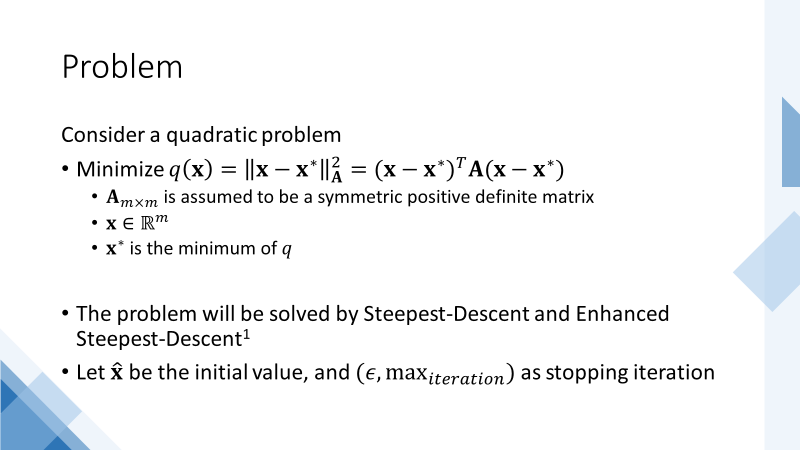## Posts

Showing posts from April, 2022

### SD-Newton-Enhanced SDEnhanced_SD_web_rev1 Comparation Steepest Decent Method, Newton Method and Enhanced Steepest Decent Method ¶ Enhanced Steepest Decent By Mohamed Kamel Riahi on "A new approach to improve ill-conditioned parabolic optimal control problem via time domain decomposition" Numer Algor (2016) 72:635-666 DOI 10.1007/s11075-015-0060-0 https://www.researchgate.net/publication/278829050_A_new_approach_to_improve_ill-conditioned_parabolic_optimal_control_problem_via_time_domain_decomposition Example 1 ¶ Consider a problem minimize : $$f(\bar{x})=x_1^2+2x_2^2+3x_3^2+x_4^2+2x_5^2+4x_6^2-x_1-x_2-x_3-x_4-x_5-x_6$$ The problem will be solved using: Steepest Decent Method Newton Method Enhanced Steepest Decent: $\hat{n}=1$, equivalent to Steepest Decent Method $\hat{n}=2$ $\hat{n}=3$ $\hat{n}=6$, equivalent to Newton Method In : import autograd.numpy as np from autograd import